×#### Thank you for registering.

One of our academic counsellors will contact you within 1 working day.

Click to Chat

1800-1023-196

+91-120-4616500

CART 0

• 0

MY CART (5)

Use Coupon: CART20 and get 20% off on all online Study Material

ITEM
DETAILS
MRP
DISCOUNT
FINAL PRICE
Total Price: Rs.

There are no items in this cart.
Continue Shopping• Complete JEE Main/Advanced Course and Test Series
• OFFERED PRICE: Rs. 15,900
• View Details

Chapter 5: Playing With Numbers Exercise – 5.3

Question: 1

Solve Cryptarithms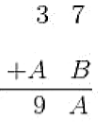Solution:

Two possible values of A are:

(i) If 7 + B < 9, 3 + A = 9

∴ A = 6

But if A = 6, 7 + B must be larger than 9.

Hence, it is impossible.

(ii) If 7 + B > 9

∴ 1 + 3 + A = 9

=> A = 5

1f A = 5 and 7 + B = 5,

B must be 8

∴ A = 5, B = 8

Question: 2

Solve Cryptarithms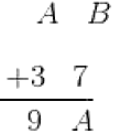Solution:

Two possibilities of A are:

(i) If B + 7 < 9,

A = 6

But clearly, if A = 6,

B + 7 > 9;

it is impossible

(ii) If B + 7 > 9,

A = 5 and B + 7 = 5

Clearly, B = 8

∴ A = 5, B = 8

Question: 3

Solve Cryptarithms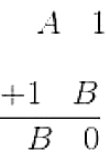Solution: 4

If 1 + B = 0 Surely, B = 9

If 1 + A + 1 = 9 Surely, A = 7

Question:

Solve Cryptarithms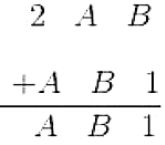Solution:

B + 1 = 8, B = 7A + B = 1, A + 7 = 1, A = 4

So, A = 4, B = 7

Question: 5

Solve Cryptarithms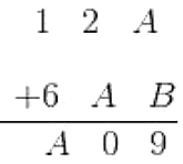Solution:

A + B = 9 as the sum of two digits can never be 192 + A = 0, A must be 8A + B = 9, 8 + B = 9, B = 1

So, A = 8, B = 1

Question: 6

Solve CryptarithmsSolution:

If A + B = 8, A + B > 9 is possible only if A = B = 9 But from 7 + B = A, A = B = 9 is impossible. Surely, A + B = 8, A + B < 9

So, A + 7 = 9, Surely A = 27 + B = A, 7 + B = 2, B = 5

So, A = 2, B = 5

Question:  7

Show that the Cryptarithm 4 × does not have any solution.

Solution:

0 is the only unit digit number, which gives the same 0 at the unit digit when multiplied by 4. So, the possible value of B is 0. Similarly, for A also, 0 is the only possible digit. But then A, B and C will all be 0, and if A, B and C become 0, these numbers cannot be of two — digit or three — digit. Therefore, both will become a one — digit number. Thus, there is no solution possible.### Course Features

• 728 Video Lectures
• Revision Notes
• Previous Year Papers
• Mind Map
• Study Planner
• NCERT Solutions
• Discussion Forum
• Test paper with Video Solution